The Lindhard Function

Hong Yao April 15, 2008

(Submitted as coursework for Physics 372, Stanford University, Spring 2008)

Response functions of a material is of vital importance to understand its physical properties and have attracted great attentions in condensed matter physics. They are intimately related to the measurements done in labs. One prototype of them is the charge response function of free electron gas, which is also known as the so-called Lindhard function. Lindhard function is famous and important partially because it can be obtained in a closed form and its analytic properties can be investigated in clarity. Moreover, even though it is for free electrons, the effect of interactions, such as Coulomb forces and electron-phonon couplings, can be in general incorporated in random phase approximation (RPA). In this study, we investigate the Lindhard function for electrons in one and three dimensions respectively (d=1, 3).

The Hamiltonian of free electron gas is given by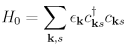where ℏ Ek= \hbar^2 \bk^2/(2m)$and$s=\uparrow,\downarrow$is the energy spectrum and spin polarization of a free electron. Here$c_{\bk s} = \frac{1}{\sqrt{L^d}} \sum_i \exp(i\br\cdot\bk) \psi_{\br s}$, in which$\psi_{\br s}$is electron annihilation operator at position$\br$with spin$s$and$L$is the linear dimension of the system. Suppose that a time-dependent external scalar potential$\phi^\textrm{ext}(\br,t) = \phi^\textrm{ext}e^{i\bq\cdot\br/\hbar-i\omega t/\hbar}$is applied to the system. The perturbative part of the Hamiltonian can be written as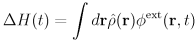where$\hat \rho(\br)\equiv -e\hat n(\br)$and$\hat n(\br)$are the electron charge and number density operator at position$\br$respectively. When the external potential is sufficiently weak, the linear response theory is applicable such that the induced charge density is proportional to the applied external scalar potential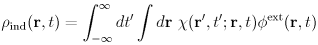where$\chi(\br,t;\br^\prime,t')$is the so-called Lindhard response function and is given by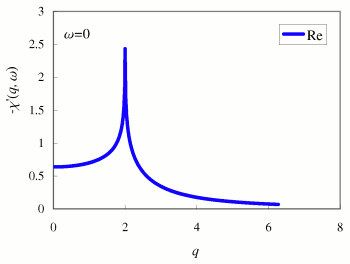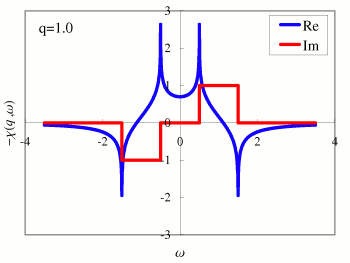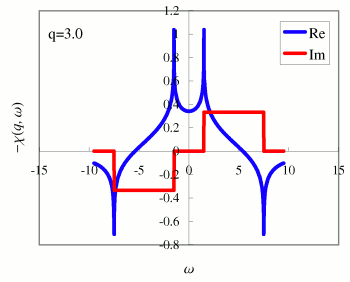Fig. 1 Lindhard function of 1D free electron gas (here we set$\hbar=e=m=k_F=1$for simplicity): (a) Real response function$\chi^\prime(q,\omega=0)$as a function of$q$. (b) Both real and imaginary response function$\chi^{\prime\prime}(q=1,\omega)$as a function of$\omega$. (c) Both real and imaginary response function$\chi^{\prime\prime}(q=3,\omega)$as a function of$\omega$.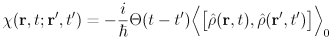where the average is respect to the system without external potential and$\hat \rho(\br,t)\equiv e^{iHt/\hbar}\rho(\br)e^{-iHt/\hbar}$. The step function$\Theta(t-t')$indicates that the response function is casual in nature. Due to the space and time translational symmetry of the unperturbed system, the response function is only a function of the relative coordinates$\br-\br^\prime$and$t-t'$:$\chi(\br,t;\br^\prime,t')\equiv \chi(\br-\br^\prime,t-t')$. The Lindhard function in momentum and frequency space is obtained by doing the following Fourier transform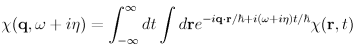where$\eta$is a infinitesimal positive number. In reality,$1/\eta$can be chosen such that it is larger than the time of measurement conducted. A related quantity to the retarded response function is the time order one, which is defined as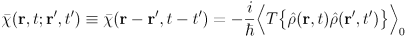where$T$is the time ordering operator which orders the operator in descending time from left to right. It turns out the retarded response function$\chi(\bq,\omega)$is related to the time ordered one$\bar\chi(\bq,\omega)$in the following way: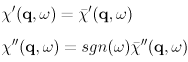where$sgn(\omega)$is the sign function of$\omega$. It is straightforward to evaluate the time ordered response function by utilizing the Wicks theorem for the ground state average of free electron gas. The analytical expression can be then obtained as follows: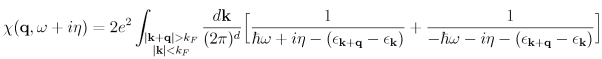where the factor of 2 is from spin polarization. The first and second term in the bracket is the resonance and anti-resonance term respectively. Note that we utilized the time reversal symmetry to write and resonance and anti-resonance term separately. The integration is limited such that$\bk+\bq$is always outside of Fermi surface and$\bk$inside. The energy cost of creating a particle-hole pair with momentum$\bq$is$\epsilon_{\bk+\bq}-\epsilon_\bk$. To evaluate the Lindhard function, it is convenient to rewrite the expression such that the integration over$\bk$can be done in a more symmetric way: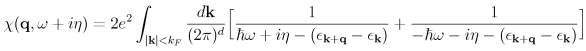where the integration is over the Fermi sea. Results for 1D electron gas In 1D, instead of a continuous Fermi surface, there are only two discrete Fermi points at$\pm k_F$. Consequently, the charge response function is quite exotic and interesting: the real part of the response function diverges when$q=2k_F$and$\omega=0$. This divergence at$q=2k_F$is responsible for the famous Peierls instability in 1D system. For simplicity, let's assumet$q>0$since the response function is symmetric in momentum if the system has the time reversal symmetry or inversion symmetry. The 1D momentum integration is straightforward. We obtain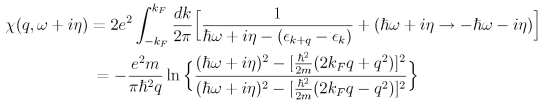where the imaginary part of a log function has branch cuts. From the resonance term, the imaginary part of the response function for position$\omega$is always negative. Similarly, from the anti-resonance term, the imaginary part of the response function for negative$\omega$is always negative. This is the rule to choose the branch of the log function. The real part and imaginary part can be obtained separately: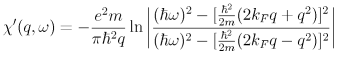and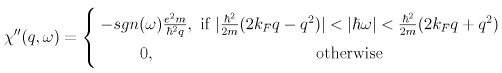where$sgn(\omega)$is the sign function. From the expression, we see that the real part of response function is even in$\omega$while the imaginary part odd in$\omega$. The energy of particle-hole pair with momentum$q$is bounded from$|\frac{\hbar^2}{2m}(2k_F q-q^2)|$to$\frac{\hbar^2}{2m}(2k_F q+q^2)$such that the imaginary part is nonzero only in this energy range. Now we are ready to plot the response function as shown in Fig. 1. In the plots, we set$\hbar=e=m=k_F=1$for simplicity. In Fig. 1a, the real response function at$\omega=0$as a function of$q$is plotted. It diverges logarithmically at$q=2k_F$. This indicates that the system is very close to an instability. Arbitrary weak electron-phonon interaction could make the uniform charge density unstable and give rise to a charge density modulation with wave length$\pi/k_F$, which is the famous Peierls instability in 1D. In Fig. 1b and 1c, both real and imaginary response functions at$q=1$and$q=3$are plotted. The real part is also divergent logarithmically at the edge of imaginary part. All the logarithmic divergence here in 1D is due to the perfect nested Fermi surface (points), which is some peculiarity of 1D systems and is generically absent in higher dimension. Results for 3D electron gas Real materials are in 3D. We define$q\equiv|\bq|$. Due to the rotational invariance of the unperturbed free electron gas, the response function depends only on the magnitude q of$\bq$but not its direction. The response function can be rewritten as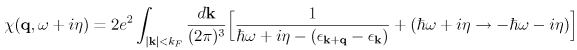which can be evaluated exactly. We obtain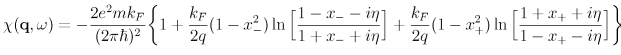where$x_\pm=\frac{m\omega}{\hbar q k_F}\pm \frac{q}{2k_F}$. As we did in 1D, the way to choose branch cut of the log function is to assign negative (positive) branch to the imaginary part when$\omega$positive (negative). Thus, we have imaginary response function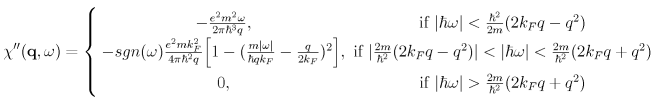where the energy of a particle-hole pair with momentum$q<2k_F$is only bounded from above. The real part of the response function is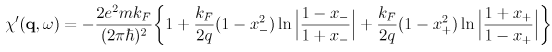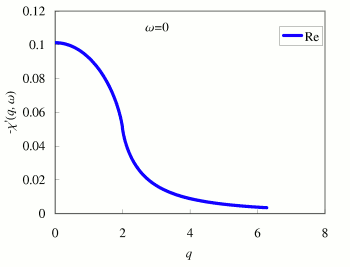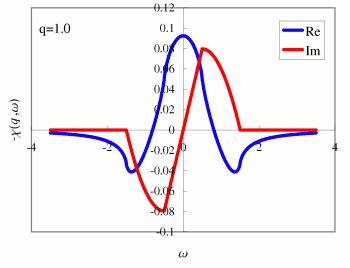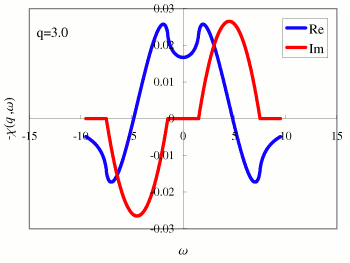Fig. 2 Lindhard function of 3D free electron gas (here we set$\hbar=e=m=k_F=1$for simplicity): (a) Real response function$\chi^\prime(q=|\bq|,\omega=0)$as a function of$q$. (b) Both real and imaginary response function$\chi^{\prime\prime}(q=1,\omega)$as a function of$\omega$. (c) Both real and imaginary response function$\chi^{\prime\prime}(q=3,\omega)$as a function of$\omega$. Using the analytic expression for the response functions, it is straightforward to plot some of them. (See Fig. 2) In Fig. 2a, the real response function at$\omega=0$as a function of$q$is plotted. It decreases continuously as$q$is creased from zero. It means that it has strongest instability at$q=0$, which corresponds to a Ferrimagnetic instability if the Coulomb repulsion is strong enough. There is no divergence at$q=2k_F$if the Fermi surface is a sphere and not nested at all. In Fig. 2b and 2c, both real and imaginary response functions at$q=1$and$q=3$are plotted. From Fig. 2b and 2c, the imaginary response function of 3D free electron gas is a continuum even for small$q$. This indicates that there is no sharp particle-part density excitation. However, this picture is not complete when the Coulomb interaction is present. The effect of the Coulomb interaction can be treated in random phase approximation (RPA) such that the sharp particle-like density excitation appears at small$q$. This collective mode is the so-called plasmon, which is first proposed by David Pines and others. At large$q$, the plasmon is damped by cloud of particle-hole pairs. concluding remarks The Lindhard function of free electron gas is so elementary that its closed form can be obtained in clarity. Despite its simplicity, some nontrivial physics manifest. In 1D, the divergence of static response function ($\omega=0\$) indicates the well known Peierls instability and the system tends to break its translational symmetry.

© 2008 H. Yao. The author grants permission to copy, distribute and display this work in unaltered form, with attribution to the author, for noncommercial purposes only. All other rights, including commercial rights, are reserved to the author.